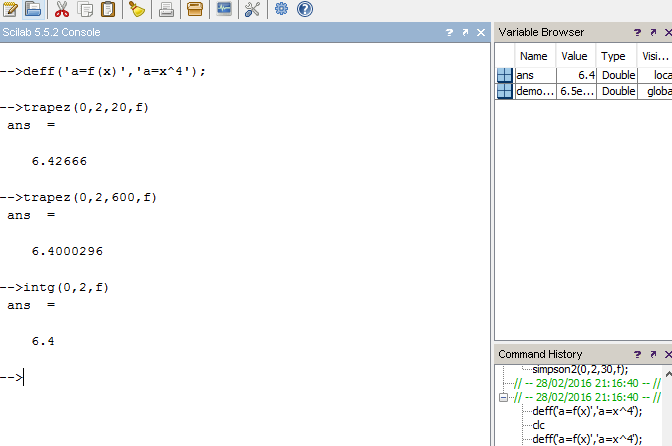# Trapezoidal Rule Integration SCILAB CODE(Program/Macro)

The following is the code for evaluating a definite integral of a given function by a Numerical Method called Trapezoidal Rule.

```//Trapezoidal Rule
//Evaluates the definite integral of a function f(x), from a to b.
//Written By: Manas Sharma(www.bragitoff.com)
funcprot(0);
function ans=trapez(a, b, n, f)//function definition of simpson
h=(b-a)/n;
sum=0;
for i=1:n-1
x=a+i*h;
sum=sum+2*f(x);
end
ans=(h/2)*(f(a)+f(b)+sum);
endfunction```

You can either copy the code above and save it as a .sci file or download the file . Once you run the code, the function ‘trapez(a,b,n,f)’ can be called by other programs or even in the console.

Function syntax:

trapez(a,b,n,f)

where,

a=initial limit(real no.)
b=final limit(real no.)
n=no. of sub-intervals(the higher the value of ‘n’ the better is the result.

Example:
The following code snippet evaluates the integral of 1/(1+x^2) from 0 to 2.[wpedon id="7041" align="center"]

## One thought on “Trapezoidal Rule Integration SCILAB CODE(Program/Macro)”

1. I have a mathlab program to convert it on scilab..I’m not able to do that can you please check once..???